Next: Regularization of the filter Up: Estimation of nonstationary PEFs Previous: Definitions

# Filter estimation

When PEFs are estimated, the matrix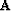is unknown. Ifis the data vector from which we want to estimate the filters, we minimize the vectoras follows: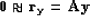(125)
which can be rewritten(126)
whereis the matrix representation of the non-stationary convolution or combination with the input vector.The transition between equations () and () is not simple. In particular, the shape of the matrixis quite different if we are doing non-stationary convolution or combination. For the convolution, we have(127)We see that for the convolution case, the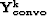matrices are diagonal operators, translating the need for one filter to be applied to one input point. The size of the matrix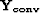iswhere nf is the number of filter coefficients. Now, for the combination, we have(128)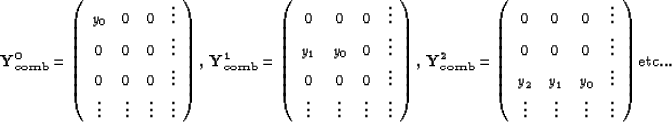We see that for the combination case, the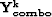matrices are row operators, translating the need for one filter to be constant for one output point. The size of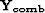is equal to the size of.For the vectorin equation () we have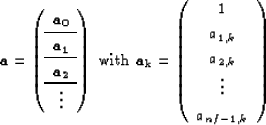(129)
where nf is the number of coefficients per filter. This definition ofis independent of.We might want to have one filter common to different input or output points instead of one filter per point. In that case, the matrixis obtained by adding successive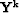matrices depending on how many points have a similar filter. Note that in the stationary case, for both the convolution and the combination case we have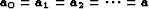and(130)
Therefore, for the matrix, we have to add all thematrices together. If we take advantage of the special structure of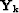for the convolution and the combination, we obtain for the stationary case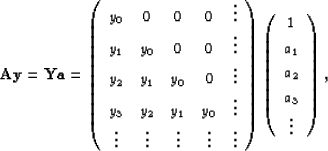(131)
which is the matrix formulation of the stationary convolution. With the definitions given in equations (), () and (), the fitting goal in equation () can be rewritten(132)
or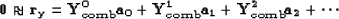(133)
Each vector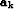has one constrained coefficient. We can then rewrite equations () and () as follows:(134)
and(135)
with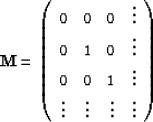(136)
The definition of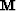assumes that the first coefficient of each filter is known. Note thatis equal for both convolution and combination methods. Having defined the matrix, we can now rewrite equation () as follows:(137)
where the square matrix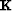is(138)
The next step consists of estimating the filter coefficients in a least-squares sense.Next: Regularization of the filter Up: Estimation of nonstationary PEFs Previous: Definitions
Stanford Exploration Project
5/5/2005